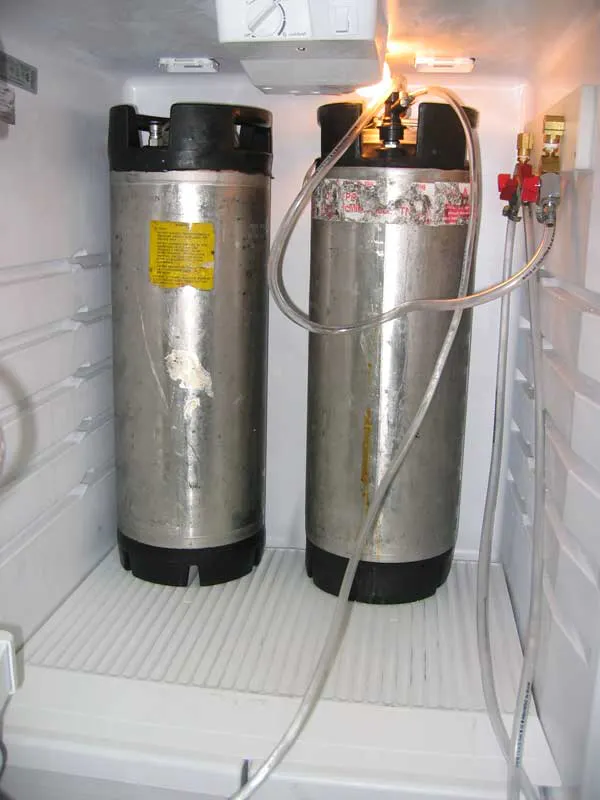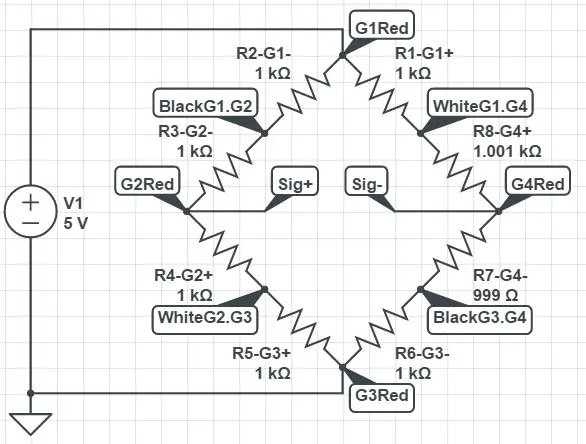# Keg Inventory Counter

This setup will give live updates over WiFi as to the amount of beer in a keg by 12oz pours!

IntermediateWork in progress6 hours1,635## Things used in this project

### Hardware componentsParticle Photon
×1
 ControlEverything.com I2C Shield
×1
 ControlEverything.com ADS1115 I2C 16-Bit Analog-Digital Converter
×1
 Digital Glass Bathroom Scale
×1

### Software apps and online servicesParticle Build Web IDE
 Mobicle
 Atomiot

### Hand tools and fabrication machines

 Wire stripper
 heat shrink connectors

## Schematics

### Full Bridge

This is a single full bridge that represents one side of the scale. The differential reading is taken at the signal points (red wires).## Code

### keg-beers.ino

Arduino
```// This #include statement was automatically added by the Particle IDE.

// CONNECT PHOTON TO ADS1115 BOARD AS FOLLOWS:
//
//  PHOTON PIN  ->  ADS11x5 PIN
//  ----------      -----------
//  3.3V        ->  VDD
//  GND         ->  GND
//  D0          ->  SDA (I2C DATA)
//  D1          ->  SCL (I2C CLOCK)
double beers_left = 0;
double beers_leftnew = 0;
int voltage = 0;
char publishString;

void setup(void)
{
Serial.begin(9600);
Serial.println("Setup..");
Particle.variable("Beers_left", &beers_left, DOUBLE);

// The ADC input range (or gain) can be changed via the following
// functions, but be careful NEVER TO EXCEED +0.3V OVER VDD ON GAINED INPUTS,
// or exceed the upper and lower limits if you adjust the input range!
//
//  *** TAKE CARE WHEN SETTING GAIN TO NOT EXCEED ABOVE LIMITS ON INPUT!!
//                                                                    -------   -------
// ads.setGain(GAIN_TWOTHIRDS);  // 2/3x gain +/- 6.144V  1 bit =       3mV       0.1875mV (DEFAULT)
// ads.setGain(GAIN_ONE);        // 1x gain   +/- 4.096V  1 bit =     2mV       0.125mV
// ads.setGain(GAIN_TWO);        // 2x gain   +/- 2.048V  1 bit =     1mV       0.0625mV
// ads.setGain(GAIN_FOUR);       // 4x gain   +/- 1.024V  1 bit =     0.5mV     0.03125mV
// ads.setGain(GAIN_EIGHT);      // 8x gain   +/- 0.512V  1 bit =     0.25mV    0.015625mV
ads.setGain(GAIN_SIXTEEN);    // 16x gain  +/- 0.256V  1 bit =     0.125mV   0.0078125mV

Serial.println("Getting differential reading from AIN0 (Positive) and AIN1 (Negative)");

}

void loop(void)
{
double multiplier = 0.0078125F; //milli Volts per bit for ADS1115
//double multiplier = 3.0F; //milli Volts per bit for ADS1105

double av0_1 = (adc0_1 * multiplier);

double av2_3 = (adc2_3 * multiplier);

float voltage = (av0_1 + av2_3);

beers_leftnew = ((voltage - .25) / 0.02109376); // The subtracted number zeros out the reading based on empty keg count. The divided number is equal to the full keg count divided by 53.3 (beers). "calibrated single beer count"

beers_left = ((beers_left * 63) + beers_leftnew) / 64;

sprintf(publishString, "%3.1f",beers_left);
Particle.publish("Beers_left",publishString);

Serial.print("AIN0_1: "); Serial.print(adc0_1); Serial.print(", AV0_1: "); Serial.print(av0_1,7); Serial.println("mV");

delay(500);

}
```

## Credits

### Eric Joyce

1 project • 1 follower
Mechanical Engineering major at UNC Charlotte, Energy concentration